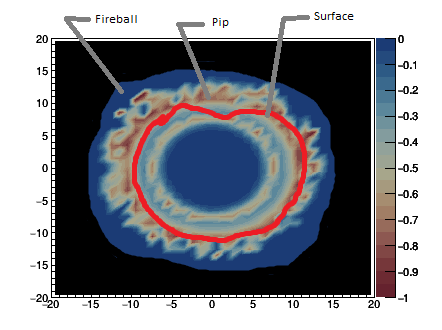# Multiple 2d graphs with different graphic options

Hello there!
I need help with some plotting. I know this might be too trivial for some of you but I struggle with it a lot.
I have four 2D graphs

``````Pip;
piT;
fireball;
surface;
``````

I successfully loaded data from the file and processed it. So I guess there is no need for posting the code but I can provide it.
Here are the graphs:

`Pip` - top left
`piT` - top right
`fireball` - bottom left
`surface` - bottom right

The `fireball` and `surface` have only 1 (non-zero) value. Let’s ignore `piT` (top right) because I would be able to figure out what to do as soon as I’ll know what to do with the `Pip` (top left).

Note: the view of the final plot would be from the top same as `Pip` and `piT` in the example

• So I’d like to plot `fireball` with one color (or two - one for fireball=1 and second for vacuum=0) [i.e. 1->Blue, 0->Black]
• Then I’d like to put `Pip` on top of that using contour, zero values wouldn’t be displayed so you can see the `fireball` area
• Also I’d like to have the z-axis of `Pip` displayed on the right next to the plot (same as in the example)
• Finally, I’d like to plot `surface` on top of that as a red circle (maybe also a little bit transparent if possible)

So the final result should look like that (grey notes not included, red possibly a little bit transparent)The only thing I know is I have to use the option `"same"` to plot more graphs but I’m even struggling to plot `fireball` and `surface` as described. This is how I made the plot on top of this post:

``````c->cd(1);
Pip[i]->Draw("CONT4Z");
c->cd(2);
piT[i]->Draw("CONT4Z");
gStyle->SetPalette(95);
c->cd(3);
fireball[i]->Draw("PFC");
c->cd(4);
surface[i]->Draw("PLC");
``````

Thanks a lot if you help me in any way!

Hi @BobekJosef; I am sure that @couet can help you with your question.

Cheers,
J.

CONT4 use special coordinates and the SAME option does not work . An intermediate canvas is needed. I guess that’s what you encounter. Here is an example showing how to make it.

``````{
TCanvas cc("cc", "cc");

TFile f("hsimple.root");
hpxpy->Draw("cont4");

Double_t BM = gPad->GetBottomMargin();
Double_t LM = gPad->GetLeftMargin();
Double_t RM = gPad->GetRightMargin();
Double_t TM = gPad->GetTopMargin();
Double_t X1 = hpxpy->GetXaxis()->GetXmin();
Double_t Y1 = hpxpy->GetYaxis()->GetXmin();
Double_t X2 = hpxpy->GetXaxis()->GetXmax();
Double_t Y2 = hpxpy->GetYaxis()->GetXmax();

null->SetFillStyle(0);
null->SetFrameFillStyle(0);
null->Draw();
null->cd();
null->Range(X1-(X2-X1)*(LM/(1-RM-LM)),
Y1-(Y2-Y1)*(BM/(1-TM-LM)),
X2+(X2-X1)*(RM/(1-RM-LM)),
Y2+(Y2-Y1)*(TM/(1-TM-LM)));

• Can I somehow do `gStyle->SetPalette(int)`; for graphs individually because it seems that it changes all graphs (something like `hpxpy->SetPalette(int)` - which doesn’t work)